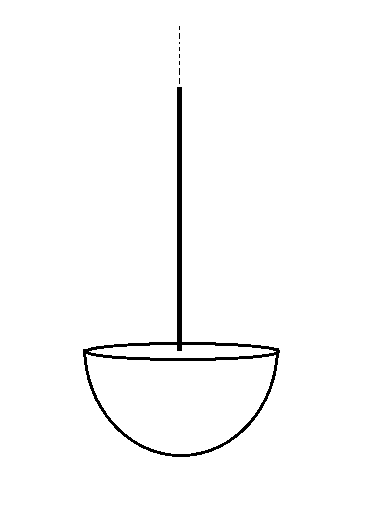# Find The Force Of Interaction

Consider a solid hemisphere having volume charge density $\rho$ and radius $R,$ and a semi-infinite rod arising from center of its base having line charge density $\lambda$. They are arranged as shown in the figure below:Find the force of interaction between them in Newtons.

Details and Assumptions (all in SI units)

• $\rho = 1.23 \times 10^{-4}$
• $\lambda = 3.21 \times 10^{-4}$
• $R = 0.5$
• $\epsilon_{0} = 8.85 \times 10^{-12}$
×